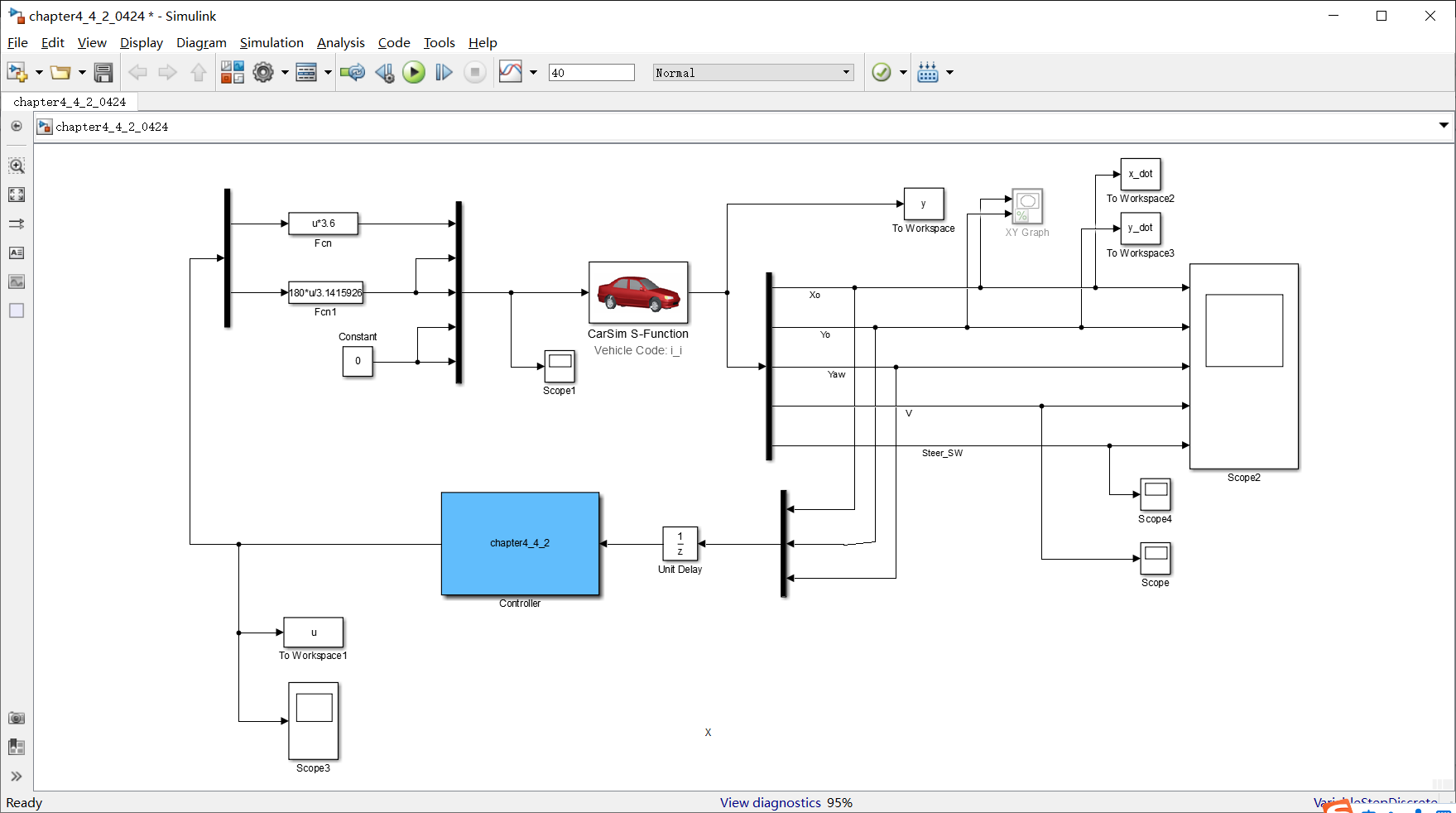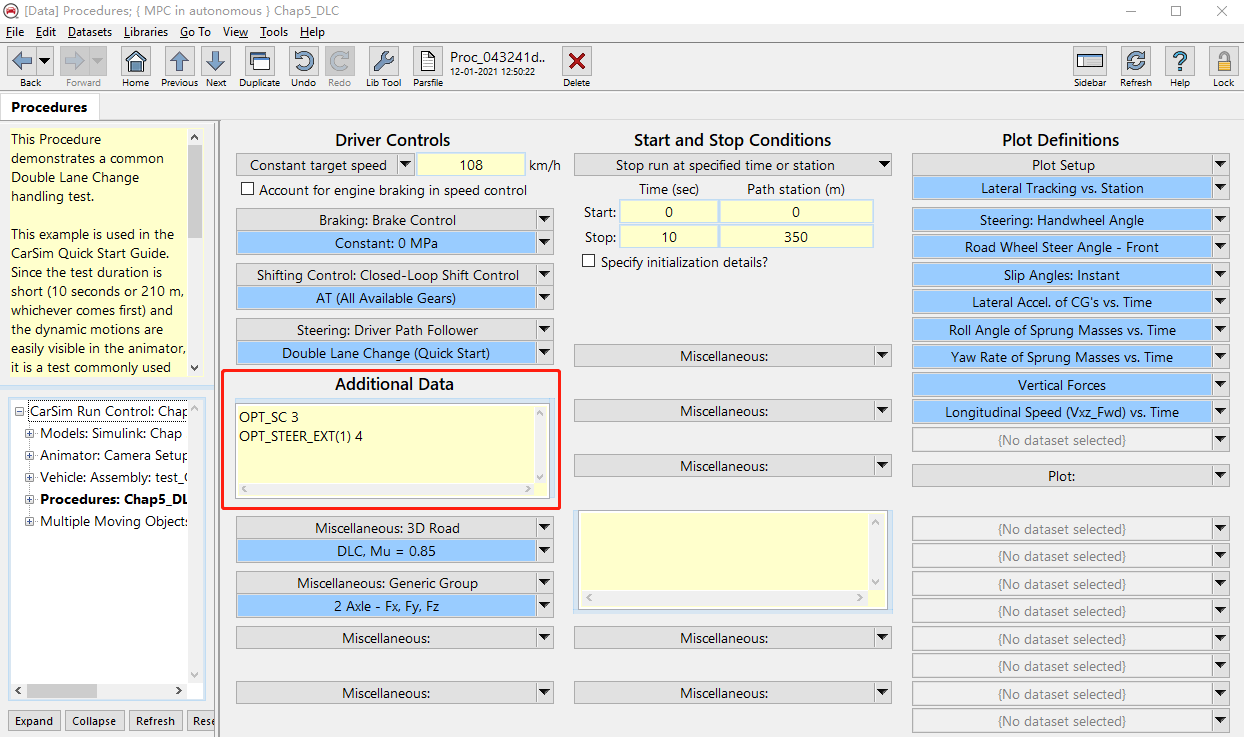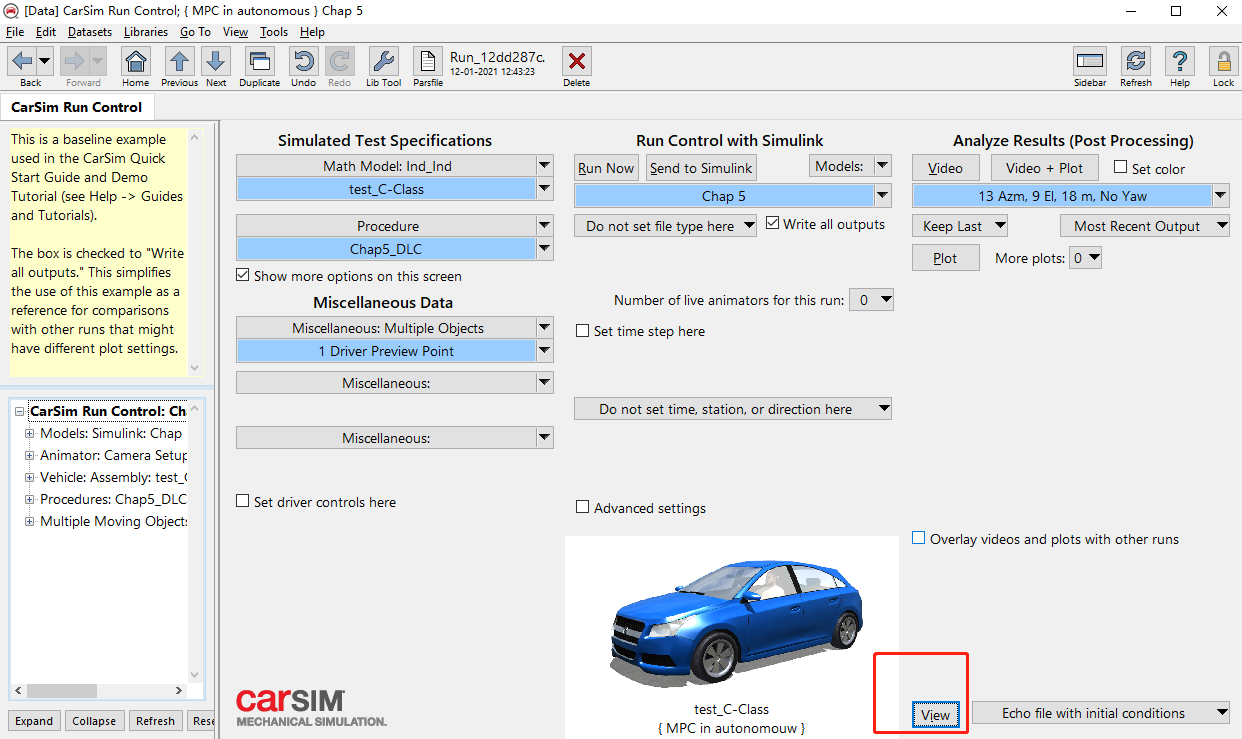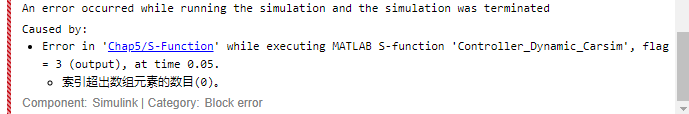• 2021-04-06 18:53:24

本代码基于无人驾驶车辆模型预测控制第一版本第五章中的代码予以纠正，下面代码已经过测试。

通过函数来生成参考轨迹（双移线），然后用模型预测控制器去跟踪。

需要会员课程的朋友可以搜索公众号：杰哥的无人驾驶便利店

点击关注并将公众号置顶，加入会员全年无限制学习后台（MPC相关矩阵的底层逻辑、纵向控制、非线性系统线性化处理及MPC算法动力学跟踪任何轨迹等）专属爆品课程（赠送会员专属全套答疑课程及全套爆品资源，且课程持续更新）！

### 代码段：

function [sys,x0,str,ts] = chapter5_2_2(t,x,u,flag)

switch flag,
case 0
[sys,x0,str,ts] = mdlInitializeSizes; % Initialization

case 2
sys = mdlUpdates(t,x,u); % Update discrete states

case 3
sys = mdlOutputs(t,x,u); % Calculate outputs

%  case 4
%   sys = mdlGetTimeOfNextVarHit(t,x,u); % Get next sample time

case {1,4,9} % Unused flags
sys = [];

otherwise
error(['unhandled flag = ',num2str(flag)]); % Error handling
end
% End of dsfunc.

%=====MPC
更多相关内容
• 本书一方面可以作为地面无人车辆、空中无人机、无人艇及移动机器人等无人车辆模型预测控制的研究资料，同时也可以作为学习模型预测控制理论的应用教材。 本书主要介绍模型预测控制理论与方法在无人驾驶车辆运动规划...
• 比一般的书籍要高清很多 作者：龚建伟 姜岩 徐威 讲述无人驾驶领域的模型预测及控制的书籍，希望能帮助到大家学习无人驾驶的相关知识。
• 无人驾驶车辆 模型预测控制》随书matlab代码。本书主要介绍模型预测控制理论与方法在无人驾驶车辆路径规划与跟踪控制方面的基础应用技术。由于模型预测控制理论数学抽象特点明显，初涉者往往需要较长时间的探索...控制理论
• 修正了错误；增加了注释；还增加了一条更复杂的参考轨迹
• 包含原书各个章节代码，可以在Matlab上运行成功，能够实现简单的路径跟踪。
• 无人驾驶车辆模型预测控制程序，程序摘之无人驾驶车辆模型预测控制书本，程序完好可运行。
• 此代码是关于无人驾驶车辆的直线轨迹跟踪模型预测控制算法实现
• 模型预测控制算法应用最经典的书籍之一，有详细的matlab代码和模型，是无人驾驶新手入门的必备书籍之一，由浅到深，看完受益匪浅
• 无人驾驶车辆 模型预测控制（龚建伟版，陈慧妍）里面MATLAB源码，有些有出入的地方，需要自己调，对于学习来说是一件很便利的事！
• 无人驾驶车辆模型预测控制,无人驾驶车辆模型预测控制 pdf,matlab源码.rar
• 无人驾驶车辆模型预测控制,无人驾驶车辆模型预测控制 pdf,matlab源码.zip
• 模型代码

function [sys,x0,str,ts] = MY_MPCController3(t,x,u,flag)
%flag为仿真过程的标志位
switch flag,%sys输出根据flag的不同而不同
case 0
[sys,x0,str,ts] = mdlInitializeSizes; % Initialization初始化

case 2
sys = mdlUpdates(t,x,u); % Update discrete states 更新离散状态

case 3
sys = mdlOutputs(t,x,u); % Calculate outputs 计算输出

case {1,4,9} % Unused flags 滞空
sys = [];

otherwise
error(['unhandled flag = ',num2str(flag)]); % Error handling
end
% End of dsfunc.

%==============================================================
% Initialization
%==============================================================

function [sys,x0,str,ts] = mdlInitializeSizes

% Call simsizes for a sizes structure, fill it in, and convert it
% to a sizes array.

sizes = simsizes; %初始化模块参数的结构体
sizes.NumContStates  = 0; %连续状态量
sizes.NumDiscStates  = 3; %离散状态量 [X,Y,PHI]
sizes.NumOutputs     = 2; %输出的个数 [speed, steering]
sizes.NumInputs      = 3; %输入量的个数 [X,Y,PHI]
sizes.DirFeedthrough = 1; % Matrix D is non-empty. 模块是否存在直接贯通（输入能直接控制输出）
sizes.NumSampleTimes = 1; %采样时间的个数
sys = simsizes(sizes);  %设置完后赋值给sys输出
x0 =[0;0;0];%状态量初始化，横纵坐标以及横摆角都为0
global U; % store current ctrl vector:[vel_m, delta_m] %store chi_tilde=[vel-vel_ref; delta - delta_ref]
U=[0;0];%初始的控制量为0 , 0两行一列
% Initialize the discrete states.
str = [];             % Set str to an empty matrix.一般在初始化中将其滞空即可
ts  = [0.1 0];       % sample time: [period, offset] 采样周期+偏移量仿真开始0s后每0.1秒运行一次
%End of mdlInitializeSizes

%==============================================================
% Update the discrete states
%==============================================================

sys = x; %更新前面算过的x状态，将x更新
%End of mdlUpdate.

%==============================================================
% Calculate outputs
%==============================================================
function sys = mdlOutputs(t,x,u)   %flag=3表示此时要计算输出，如果sys=[],则表示没有输出
global a b u_piao; %a,b和u_piao矩阵为全局矩阵
global U; %store chi_tilde=[vel-vel_ref; delta - delta_ref]
global kesi;%kesi为全局，新的状态向量，[k时刻的状态量误差；k-1时刻的控制量误差],5*1矩阵（误差即偏差）

tic
Nx=3;%状态量的个数
Nu =2;%控制量的个数
Np =60;%预测步长
Nc=30;%控制步长
Row=10;%松弛因子
fprintf('Update start, t=%6.3f\n',t)%u(1)为X；u(2)为Y
t_d = u(3)*3.1415926/180;% t_d为横摆角，CarSim输出的为角度，角度转换为弧度。u(3)是车辆的实际航向角

%    %直线路径
%     r(1)=5*t; %ref_x-axis
%     r(2)=5;%ref_y-axis
%     vd1=5;% ref_velocity
%     vd2=0;% ref_steering

%半径为25m的圆形轨迹, 圆心为(0, 35), 速度为5m/s
r(1)=25*sin(0.2*t); %参考的X
r(2)=35-25*cos(0.2*t);%参考的Y
r(3)=0.2*t; %  参考的横摆角，w = (v/r) = (vr*tan(cita))/l = 0.2
vd1=5; %速度5m/s，这是参考速度
vd2=0.104; %前轮偏角=(轴距/半径）=(2.6/25)=0.104，这是参考前轮转角

%     %半径为25m的圆形轨迹, 圆心为(0, 35), 速度为3m/s
%     r(1)=25*sin(0.12*t);
%     r(2)=25+10-25*cos(0.12*t);
%     r(3)=0.12*t;
%     vd1=3;
%     vd2=0.104;
%半径为25m的圆形轨迹, 圆心为(0, 35), 速度为10m/s
%      r(1)=25*sin(0.4*t);
%      r(2)=25+10-25*cos(0.4*t);
%      r(3)=0.4*t;
%      vd1=10;
%      vd2=0.104;
%     %半径为25m的圆形轨迹, 圆心为(0, 35), 速度为4m/s
%      r(1)=25*sin(0.16*t);
%      r(2)=25+10-25*cos(0.16*t);
%      r(3)=0.16*t;
%      vd1=4;
%      vd2=0.104;

kesi=zeros(Nx+Nu,1); %构造新的状态量矩阵 ，5*1维矩阵
kesi(1) = u(1)-r(1);%u(1)==X(1),x_offset    u（1）是指纵向位置x;  r(1)是指参考的纵向位置
kesi(2) = u(2)-r(2);%u(2)==X(2),y_offset    u(2)是指横向位置；r(2)是指参考的横向位置。
kesi(3) = t_d - r(3); %第三行是横摆角的误差。t_d是指车辆的横摆角；r(3)是指参考的横摆角。
kesi(4)=U(1); % vel-vel_ref 上一时刻的速度误差
kesi(5)=U(2); % steer_angle - steering_ref 上一时刻前轮偏角误差
fprintf('vel-offset=%4.2f, steering-offset, U(2)=%4.2f\n',U(1), U(2))

T=0.1;%采样时间为0.1s
T_all=40;%临时设定，总的仿真时间。设置总的仿真时间防止仿真溢出。
% Mobile Robot Parameters
L = 2.6; % wheelbase of carsim vehicle 轴距为2.6m
% Mobile Robot variable

%矩阵初始化
u_piao=zeros(Nu,1); %构造3*2维度（认为这应该是Nu=2*1维度）的矩阵来存放控制量误差的变化量
%u_piao=zeros(Nx,Nu);%构造3*2维度的矩阵来存放控制量误差
Q=100 * eye(Nx*Np,Nx*Np);  %权重矩阵Q为180*180的单位矩阵，Q是指输出状态矩阵
R=5*eye(Nu*Nc); %权重矩阵R为60*60的单位矩阵。R是指控制量误差的状态矩阵
%R=5*eye(Nu*Nc); %权重矩阵R为60*60的单位矩阵
t_d1 = r(3);%这里应该是参考值  原代码写的是实际输出的侧偏角（有误）
a=[1    0   -vd1*sin(t_d1)*T;
0    1   vd1*cos(t_d1)*T;
0    0   1;]; %a为我们线性离散化后的第一个系数矩阵
b=[cos(t_d1)*T        0;
sin(t_d1)*T        0;
tan(vd2)*T/L      vd1*T/(cos(vd2)^2);];%b为我们线性离散化后的第二个系数矩阵

%预测方程的A,B
A_cell=cell(2,2); % 构建2*2的元胞数组
B_cell=cell(2,1); %构建2*1的元胞数组
A_cell{1,1}=a; %将a矩阵放到A_cell的第一行第一个位置
A_cell{1,2}=b; %将b矩阵放到A_cell的第一行第二个位置
A_cell{2,1}=zeros(Nu,Nx); %将2*3的零矩阵放到A_cell第二行的第一个位置
A_cell{2,2}=eye(Nu); %将2*2的单位阵放到A_cell第二行的第二个位置
B_cell{1,1}=b; %将b矩阵放到B_cell的第一行
B_cell{2,1}=eye(Nu); %将2*2的单位阵放到B_cell第二行
A=cell2mat(A_cell); %这里的A就是我们在推导下一时刻的状态空间时候的A
B=cell2mat(B_cell); %这里的B就是我们在推导下一时刻的状态空间时候的B
C=[ 1 0 0 0 0;
0 1 0 0 0;
0 0 1 0 0];%这个C矩阵是我们输出方程yita的系数矩阵，因为我们可能不需要把每个状态量都输出所以就可以通过设置C矩阵来输出我们想要的状态量，在这里我们输出的是X的误差、Y的误差以及横摆角的误差

PHI_cell=cell(Np,1);%这个PHI是我们通过总结规律得到的等式右边的第一个系数矩阵，60*1维度
THETA_cell=cell(Np,Nc);%这里的THETA为我们通过总结规律的到的等式右边的第二个系数矩阵，60*30维度
for j=1:1:Np
PHI_cell{j,1}=C*A^j; %通过循环来给第一个系数矩阵赋值
for k=1:1:Nc
if k<=j
THETA_cell{j,k}=C*A^(j-k)*B; %C为3*5矩阵；A为5*5；B为5*2，所以C*A*B为3*2矩阵
else
THETA_cell{j,k}=zeros(Nx,Nu);
end
end
end
PHI=cell2mat(PHI_cell);%size(PHI)=[Nx*Np Nx+Nu] 180*5维度
THETA=cell2mat(THETA_cell);%size(THETA)=[Nx*Np Nu*(Nc+1)]

H_cell=cell(2,2); %这里的H为我们二次规划中的H矩阵，以下来构造二次规划中的H矩阵
H_cell{1,1}=THETA'*Q*THETA+R;
H_cell{1,2}=zeros(Nu*Nc,1); %60*1维度
H_cell{2,1}=zeros(1,Nu*Nc); %1*60维度
H_cell{2,2}=Row; %H矩阵的右下角的元素就只有一个就是我们的松弛因子
H=cell2mat(H_cell);%由于松弛因子的影响，最终的H矩阵为61*61
H=(H+H')/2;

error=PHI*kesi; %这里的error就是我们所设的E矩阵
f_cell=cell(1,2);%f为二次规划的第二个向量，下面我们来构造它
f_cell{1,1} = 2*(error'*Q*THETA);
f_cell{1,2} = 0;
f=cell2mat(f_cell);%将元胞数组转化为矩阵

%% 以下为约束生成区域
%不等式约束
A_t=zeros(Nc,Nc);%见falcone论文 P181
for p=1:1:Nc
for q=1:1:Nc
if q<=p
A_t(p,q)=1;
else
A_t(p,q)=0;
end
end
end
A_I=kron(A_t,eye(Nu));%对应于falcone论文约束处理的矩阵A,求克罗内克积
Ut=kron(ones(Nc,1), U);%此处的U表示的应该是上一时刻控制量误差（即偏差）组成的矩阵。
umin=[-0.2;  -0.54];%[min_vel, min_steer]维数与控制变量的个数相同
umax=[0.2;   0.332]; %[max_vel, max_steer],%0.436rad = 25deg
delta_umin = [-0.05;  -0.0082]; % 0.0082rad = 0.47deg
delta_umax = [0.05;  0.0082];
Umin=kron(ones(Nc,1),umin);
Umax=kron(ones(Nc,1),umax);

%二次规划不等式约束 Ax <= b
A_cons_cell={A_I zeros(Nu*Nc, 1); -A_I zeros(Nu*Nc, 1)};
b_cons_cell={Umax-Ut;-Umin+Ut};
A_cons=cell2mat(A_cons_cell);%（求解方程）状态量不等式约束增益矩阵，转换为绝对值的取值范围
b_cons=cell2mat(b_cons_cell);%（求解方程）状态量不等式约束的取值

% 状态量约束
M = 10;%松弛因子上限
delta_Umin = kron(ones(Nc,1),delta_umin);
delta_Umax = kron(ones(Nc,1),delta_umax);
lb = [delta_Umin; 0];%（求解方程）状态量下界,包含控制时域内控制增量和松弛因子下界
ub = [delta_Umax; M];%（求解方程）状态量上界，包含控制时域内控制增量和松弛因子上界M

%% 开始求解过程
%     options = optimset('Algorithm','active-set');
options = optimset('Algorithm','interior-point-convex');
[X, fval,exitflag]=quadprog(H, f, A_cons, b_cons,[], [],lb,ub,[],options);

%% 计算输出
u_piao(1)=X(1);% X1为二次规划求出来的是控制时域内最优的速度控制增量
u_piao(2)=X(2);% X2为二次规划求出来的最优前轮转角控制增量
U(1)=kesi(4)+u_piao(1);%用于存储上一个时刻的控制量  %kesi4为速度误差。kesi4为速度误差与速度误差增量u_piao(1)相加得到我们下一时刻的kesi矩阵的第四行
U(2)=kesi(5)+u_piao(2);%kesi5为k-1时刻前轮偏角误差，道理同上，与前轮偏角误差的增量相加得到我们下一时刻的kesi矩阵的第五行
u_real(1) = U(1) + vd1;%再与下面的参考速度相加才是真正的速度
u_real(2) = U(2) + vd2;%道理同上

sys= [u_real(1); u_real(2)]; % vel, steering, x, y 将算出来的真正的控制量输出输出
toc
% End of mdlOutputs.

展开全文经验分享
• 无人驾驶车辆模型预测控制第五章修改

# 第五章 5.2主动转向控制的联合仿真

运行环境

• CarSim 2020
• Matlab 2020a
由于carsim2020较carsim8.0有较大版本变动，在进行前轮偏角进行控制时，此时需要加上指令。
OPT_SC 3 通过该指令激活速度控制车辆；
opt_steer_ext(1) 4 通过该指令激活前轮转角控制车辆；
以上指令需要在 Drive Controls界面中修改，具体如下图：具体可以在主界面右下角通过VIEW看到具体含义：在与Simulink进行联合仿真时需要注意，求解时问题约束应进行简化，便于求解，不然会由于无可行解（参见：北理工无人驾驶车辆模型预测控制第4、5章代码勘误），同时还需对二次规划中的权重矩阵H进行修改（参见：《无人驾驶车辆模型预测控制第一版》第五章代码（下））具体错误如下：
Error in 'Chap5/S-Function' while executing MATLAB S-function 'Controller_Dynamic_Carsim', flag = 3 (output), at time 0.05. 索引超出数组元素的数目(0)。其它按照书中配置应该就没有其它问题了，控制效果主要可以通过修改采样时间以及预测、控制时域以及权重矩阵等，由于我的目的不在于此，就没有仔细调调整了。

展开全文• 无人驾驶车辆模型预测控制》3.3.3工程实例 主要针对《无人驾驶车辆模型预测控制》这本书的第三章3.3.3的工程实例进行详细的代码分析，结合自己在学习以及推导的MPC算法的一些理解。 书中源代码 以无人驾驶车辆...

# 《无人驾驶车辆模型预测控制》3.3.3工程实例

• 主要针对《无人驾驶车辆模型预测控制》这本书的第三章3.3.3的工程实例进行详细的代码分析，结合自己在学习以及推导的MPC算法的一些理解。

## 书中源代码

• 以无人驾驶车辆的轨迹跟踪问题作为应用的背景，无人驾驶车辆在一个给定的位置出发，通过离散轨迹点（本工程实例使用的是离散的轨迹点）或者连续轨迹函数的指引，最终跟上期望的轨迹。
• 轨迹跟踪仿真基本设定：车辆从坐标原点出发，以期望纵向速度v=1m/s跟踪一条直线轨迹y=2。采样时间为0.05s（50ms），仿真的总时间设定为5s，在Matab中进行仿真。控制的目标是无人驾驶车辆在低速情况下的跟踪控制，因此考虑使用车辆的运动学方程作为预测模型。通过将车辆的运动学方程进行线性化（还有离散化的处理），得到线性时变模型。
%% 生成目标轨迹
N = 100; % 目标轨迹点的数量
T = 0.05; % 采样周期
Xout = zeros(N,3); % Xout用于存储目标轨迹点上的车辆状态；车辆共有3个状态变量：X轴坐标、Y轴坐标、航向角φ；Xout就是用来表示期望轨迹上车辆的状态
Tout = zeros(N,1); % Tout用于存储离散的时间序列
for k = 1:1:N
Xout(k,1) = k*T; % 设置目标轨迹点的X轴坐标
Xout(k,2) = 2; % 设置目标轨迹点的Y轴坐标；此仿真实例轨迹是一条直线y=2
Xout(k,3) = 0; % 设置目标轨迹点的航向角;此仿真实例中由于轨迹是一条直线，所以车辆的航向角也是固定的，为0
Tout(k,1) = (k-1)*T; % 由此可见，目标轨迹点对应的时刻，和采样时刻保持一致；整个仿真时长为100*0.05共计5秒（默认单位时长为1秒）
% 由上可知，车辆在目标轨迹上，每两个轨迹点间的X轴的坐标相差0.05，每两个轨迹点对应的时刻相差0.05，因此车辆在X轴上的速度为1m/s
end


代码第一部分主要是根据仿真要求设定的一些基本的情况，参考轨迹后面可以自己进行修改，比如圆形轨迹或者8字形的的轨迹本文的后面还有对轨迹进行修改的一个测试

%% 描述系统的基本情况
Nx = 3; % 状态量共有3个：X轴坐标、Y轴坐标、航向角φ；
Nu = 2; % 控制量共有2个：车辆的纵向速度v（后轴速度），前轮偏角δ

[Nr,Nc] = size(Xout); % 获取目标轨迹点车辆状态矩阵的维度，Nr代表目标点的数目100，Nc代表车辆状态的数目3；即100行3列
Tsim = 20; % MPC的预测时域，即当车辆处于时刻k时，对k+1,k+2,k+3,...,k+Tsim时刻的车辆状态进行预测
X0 = [0 0 pi/3]; % 车辆初始状态，X轴坐标为0，Y坐标为0，航向角pi/3
L = 1; % 车辆轴距
vd1 = 1; % 车辆的目标纵向速度v=1m/s
vd2 = 0; % 车辆的目标前轮偏角δ=0


代码的第二部分也没有什么好说的，也是一些车辆基本情况和MPC预测时域的设定

%% 定义相关矩阵
% x_real矩阵，用于存储每一个仿真时刻，车辆的实际位置状态，初始状态为上面定义的X0
x_real = zeros(Nr,Nc); %生成一个用来存储每一个仿真时刻车辆实际位置的空矩阵
x_real(1,:) = X0;%将车辆初始时刻的状态加入x_real
% x_piao矩阵，用于存储每一个仿真时刻，车辆的实际位置状态与目标位置状态之间的偏差
x_piao = zeros(Nr,Nc); %同样地，生成一个用来存储每一个仿真时刻车辆的实际位置状态与目标位置状态的偏差
x_piao(1,:) = x_real(1,:)-Xout(1,:);%车辆初始时刻所在的位置状态与目标位置状态之间的偏差
% X_PIAO的每一行代表一个仿真时刻（对应一个轨迹点）
% 在每一个仿真时刻，都需要对车辆未来20（Tsim）个时刻的实际位置状态与目标位置状态的偏差进行预测，所以X_PIAO每一行的数据可以分为20（Tsim）个组
% 每一组有3（Nx）列，分别对应车辆3个状态的偏差；100行，20X3列
X_PIAO = zeros(Nr,Nx*Tsim);%生成一个用来存储在每一个仿真时刻预测未来20（Tsim）个时域车辆与目标轨迹之间的状态偏差
% u_real矩阵，用于存储每一个仿真时刻，车辆的实际控制量（实际运动状态）（纵向速度、前轮偏角）
u_real = zeros(Nr,Nu);%100行2列
% u_piao矩阵，用于存储每一个仿真时刻，车辆的实际控制量（实际运动状态）与目标控制量（运动状态）之间的偏差
u_piao = zeros(Nr,Nu);%同样100行2列
% XXX的每一行代表一个仿真时刻（对应一个轨迹点）
% 在每一个仿真时刻，都需要对未来20个时刻的状态值进行预测，所以XXX每一行的数据可以分为20（Tsim）个组
% 每一组有3（Nx）列，分别对应车辆3个状态
XXX = zeros(Nr,Nx*Tsim);% 用于保存每个时刻预测的所有状态值100行60列
% q为加权矩阵，用于调节每个状态量（X轴坐标、Y轴坐标、航向角）在目标函数中比重（权重系数）
q = [1 0 0;0 1 0;0 0 0.5];
Q_cell = cell(Tsim,Tsim); % 元组Q_cell的作用是，将q作用于预测的每一个点上（共计Tsim个点）
for i = 1:1:Tsim
for j = 1:1:Tsim
if i == j
Q_cell{i,j}=q;%将加权矩阵q赋给二维元胞数组行列索引位置相等处的数组元素
else
Q_cell{i,j}=zeros(Nx,Nx);%其他的位置都赋给0加权矩阵
end
end
end
Q = cell2mat(Q_cell); % 通过cell2mat()函数将元胞数组转化成最终的状态加权矩阵Q，调节每个预测点上，三个状态量各自在目标函数中比重；20X3行20X3列
R = 0.1*eye(Nu*Tsim,Nu*Tsim); % 最终的控制量加权矩阵，用于调节控制量偏差在目标函数中的比重


代码的第三部分是对相关矩阵的定义，其中XXX表示存储在每个仿真时刻预测的未来20个时刻的状态值这部分表示的含义需要进一步理解

%% 模型预测控制的主体函数
%在每一个仿真时刻，模型预测控制是如何进行预测、滚动优化以及反馈矫校正
for i = 1:1:Nr
t_d = Xout(i,3); % t_d为i时刻的期望航向角
% 下一预测点的状态偏差 = a*当前点的状态偏差 + b*当前点的控制量偏差   （状态偏差，即车辆位置偏差；控制量偏差，即车辆运动偏差）
a = [1 0 -vd1*sin(t_d)*T;   % 矩阵a，是关于目标车速和目标航向角的函数；
0 1 vd1*cos(t_d)*T; % 由于目标车速和目标航向角保持恒定
0 0 1;]; % 该仿真中给出的期望车速和航向都是不变的，所以，矩阵a恒定不变
b = [cos(t_d)*T 0; % 与矩阵a相似，矩阵b也保持恒定
sin(t_d)*T 0;
tan(vd2)*T/L vd1*T/(L*cos(vd2)^2);];% 此处已经对书中的错误进行了更正，原书中给出的代码为：vd2*T/L vd1*T/(cos(vd2)^2);使用了近似
% 目标函数，是预测时域内预测点与目标点的方差之和；  预测时域为Tsim，即存在20个预测点；  预测点与目标点方差之和为“状态方差（位置方差）” + “控制量方差（运动方差）”；
A_cell = cell(Tsim,1);%A_cell为预测模型状态方差的系数矩阵
B_cell = cell(Tsim,Tsim);B_cell为预测模型控制量方差的系数矩阵
for j = 1:1:Tsim
% 因为目标速度vd1、目标航向角φ保持不变，所以A(k|k)、A(k+1|k)、...A(k+Tsim|k)全都相同
A_cell{j,1} = a^j;
for k = 1:1:Tsim
if k<=j
B_cell{j,k} = (a^(j-k))*b;
else
B_cell{j,k} = zeros(Nx,Nu);
end
end
end
%通过cell2mat()函数将A_cell和B_cell分别转化成矩阵形式，得到预测模型的两个重要系数矩阵
A = cell2mat(A_cell);%60行3列
B = cell2mat(B_cell);%60行40列
H = 2*(B'*Q*B + R);%根据二次规划的标准型，定义相关的矩阵
f = 2*B'*Q*A*x_piao(i,:)';
A_cons = [];
b_cons = [];
%对两个控制量偏差进行约束，分别定义速度偏差和前轮转向偏差的上下限
% 每一步， -2.2 <= v - vr <= 0.2
% 每一步， -0.64 <= 前轮偏角 - 目标前轮偏角 <= 0.64
ub = [0.2; 0.64];    lb = [-2.2; -0.64];
% 通过二次规划求解，得到的X，为最优控制偏差矩阵[Dlt_u,Dlt_u1,Dlt_u2,Dlt_u3,...,Dlt_u19]
% 通过运动学公式，计算得到在控制偏差矩阵[Dlt_u,Dlt_u1,Dlt_u2,Dlt_u3,...,Dlt_u19]作用下的状态偏差矩阵
% [Dlt_x_1,Dlt_x_2,Dlt_x_3,Dlt_x1_1,Dlt_x2_1,Dlt_x3_1, Dlt_x1_2,Dlt_x2_2,Dlt_x3_2, ..., Dlt_x1_19,Dlt_x2_19,Dlt_x3_19]
% 并将其存储在 X_PIAO(i,:) 中
X_PIAO(i,:) = (A*x_piao(i,:)'+B*X)';%1行60列

% 下面的j，虽然是上面for循环的变量，但是MATLAB不会将j自动清零；所以，j的大小即为Tsim(20)
if i+j < Nr % i代表当前时刻，j代表相对于当前时刻向前预测的Tsim时刻；此句代表预测的时刻没有超出整个仿真时长
for k = 1:1:Tsim
% 存储预测到的未来Tsim（20）个时域内状态值（预测值 = 在最优控制偏差下得到的状态偏差值 + 目标状态值
XXX(i,1+3*(k-1)) = X_PIAO(i,1+3*(k-1)) + Xout(i+k,1);
XXX(i,2+3*(k-1)) = X_PIAO(i,2+3*(k-1)) + Xout(i+k,2);
XXX(i,3+3*(k-1)) = X_PIAO(i,3+3*(k-1)) + Xout(i+k,3);
end
else
%如果预测时域超出了整个的仿真的时长，预测的状态都是相对目标点的
for k = 1:1:Tsim
XXX(i,1+3*(k-1)) = X_PIAO(i,1+3*(k-1)) + Xout(Nr,1);
XXX(i,2+3*(k-1)) = X_PIAO(i,2+3*(k-1)) + Xout(Nr,2);
XXX(i,3+3*(k-1)) = X_PIAO(i,3+3*(k-1)) + Xout(Nr,3);
end
end
%分别取最优控制序列的第一个元素，包括纵向速度和前轮转向偏角；将他们分别赋给u_piao，
%即将这个最优解作为当前时刻车辆实际的控制量与目标控制量之间的控制量偏差
u_piao(i,1) = X(1,1);
u_piao(i,2) = X(2,1);

Tvec = [0:0.05:4];%定义的这个矩阵是何意？
x00 = x_real(i,:); % x00为当前时刻车辆的位置状态
vd11 = vd1 + u_piao(i,1); % 依据MPC求得的最优控制偏移u_piao(i,1)，以及当前的控制量vd1，计算出下一时刻的控制量vd11
vd22 = vd2 + u_piao(i,2); % 依据MPC求得的最优控制偏移u_piao(i,2)，以及当前的控制量vd2，计算出下一时刻的控制量vd22
% 知道了当前时刻的位置状态、运动状态（控制量）
% 下面根据运动学方程，求解下一时刻车辆的位置状态
%下面是求解的微分方程
XOUT = dsolve('Dx - vd11*cos(z) = 0',...
'Dy - vd11*sin(z) = 0',...
'Dz - vd22 = 0',...
'x(0) = x00(1)',...
'y(0) = x00(2)',...
'z(0) = x00(3)');
t = T; % T为采样周期
x_real(i+1,1) = eval(XOUT.x);
x_real(i+1,2) = eval(XOUT.y);
x_real(i+1,3) = eval(XOUT.z);
if (i<Nr)
x_piao(i+1,:) = x_real(i+1,:) - Xout(i+1,:);
end
% 根据目标运动状态（目标速度和目标前轮转角），以及上述求得的最优控制偏差（运动量偏差）
% 计算当前最佳的控制量（当前速度、当前转向角）
u_real(i,1) = vd1 + u_piao(i,1); % vd1为目标控制量：目标速度
u_real(i,2) = vd2 + u_piao(i,2); % vd1为目标控制量：目标前轮转向角

figure(1);
plot(Xout(1:Nr,1),Xout(1:Nr,2));
hold on;
plot(x_real(i,1),x_real(i,2),'r *')
xlabel('X[m]');
axis([-1 5 -1 3]);
ylabel('Y[m]');
hold on;
%绘制未来Tsim时域内预测的车辆的状态（黄线）
for k = 1:1:Tsim
X(i,k+1) = XXX(i,1+3*(k-1));
Y(i,k+1) = XXX(i,2+3*(k-1));
end
X(i,1) = x_real(i,1);
Y(i,1) = x_real(i,2);
plot(X(i,:),Y(i,:),'y')
hold on
end


第四部分的代码也是代码的主要核心的部分，需要多次反复的来阅读理解。。。最后绘制了figure(1)将车辆在MPC作用下跟踪参考轨迹的过程表现了出来

%系统状态量随时间变化
figure(2)
subplot(3,1,1);
plot(Tout(1:Nr),Xout(1:Nr,1));
hold on;
plot(Tout(1:Nr),x_real(1:Nr,1),'r');
xlabel('采样时间T');
ylabel('横向位置X')%绘制了状态量X随着时间的变化（红线是车辆实际的状态量X）
subplot(3,1,2);
plot(Tout(1:Nr),Xout(1:Nr,2));
hold on;
plot(Tout(1:Nr),x_real(1:Nr,2),'r');
xlabel('采样时间T');
ylabel('纵向位置Y')%绘制了状态量Y随时间的变化（红线是车辆实际的状态量Y）
subplot(3,1,3);
plot(Tout(1:Nr),Xout(1:Nr,3));
hold on;
plot(Tout(1:Nr),x_real(1:Nr,3),'r');
hold on;
xlabel('采样时间T');
ylabel('\theta')%绘制了状态量φ随时间的变化（红线是车辆的实际状态量φ）


第五部分的代码主要是绘制了车辆的实际状态量和目标状态量随着时间的变化

figure(3)
subplot(2,1,1);
plot(Tout(1:Nr),u_real(1:Nr,1),'r');%绘制车辆实际速度随时间变化的曲线
xlabel('采样时间T');
ylabel('纵向速度')
subplot(2,1,2)
plot(Tout(1:Nr),u_real(1:Nr,2),'r');绘制车辆前轮偏角随时间变化的曲线
xlabel('采样时间T');
ylabel('角速度')%#####这里应该不是指的角速度而是指前轮（方向盘）转角吧


第六部分的代码绘制了车辆的两个控制量（速度v和前轮转角δ）随着时间的变化曲线

figure(4)
subplot(3,1,1);
plot(Tout(1:Nr),x_piao(1:Nr,1));
xlabel('采样时间T');
ylabel('e(x)');%绘制车辆实际状态量X与参考状态量X之间的偏差曲线
subplot(3,1,2);
plot(Tout(1:Nr),x_piao(1:Nr,2));
xlabel('采样时间T');
ylabel('e(y)');%绘制车辆实际状态量Y与参考状态量Y之间的偏差曲线
subplot(3,1,3);
plot(Tout(1:Nr),x_piao(1:Nr,3));
xlabel('采样时间T');
ylabel('e(\theta)');%绘制车辆实际状态量φ与参考状态量φ之间的偏差曲线


第七部分的代码绘制了车辆当前实际的三个状态量与目标状态量之间的偏差，至此，整个的程序结束。

展开全文• 本书主要介绍模型预测控制理论与方法在无人驾驶车辆路径规划与...本书一方面可以作为地面无人车辆、空中无人机、无人艇及移动机器人等无人车辆模型预测控制的研究资料，同时也可以作为学习模型预测控制理论的应用教材。
• 北理工无人驾驶车辆模型预测控制第4、5章代码勘误 以下内容为实际使用过程中发现的小问题，不代表原代码逻辑错误。 第4章 程序约束生成区域中 delta_umin=[0.05;-0.0082;]; 应改为： delta_umin=[-0.05;-0.0082;...matlabMPC
• 一些概念 路径跟踪： 跟踪reference path即可，不受时间约束。...模型预测控制与运动规划和控制： 低速：运动学特性大 高速：动力学特性影响大 基于简化的动力学模型与运动学模型的模型预测控制是轨迹跟踪研究热点 ......